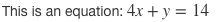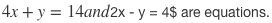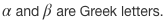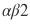# Math Mode on QuizalizeWriting equations, fractions or other complex formulas on a computer can be a terribly dull affair. There are a few words with which to describe the equation editor in Microsoft Word, but none are appropriate for audiences of all ages!

Thankfully, Quizalize features a super simple and quick way to include good-looking formulas in your questions and answers. Here’s an example:All you need to do is to click the Math Mode button, in the upper right corner. The question input screen will then show live preview boxes. As you enter your formulas in the super-straightforward LaTex format (more on this below), these boxes automatically update so you can easily check your work.Quizalize is built to handle LaTex format equations. It’s actually rather simple. All you need to do is include your formula in between two $symbols. Here’s a quick example. Writing this: This is an equation:$4x + y = 14$Turns into this:As you can see, the text in between the dollar symbols was transformed into a nice-looking equation. The rest of the text was not. It is important to remember to include the second$, otherwise it will not work.

It is possible to include 2 or more separate equations with text in-between. All you need to do is remember to enclose each part with $symbols. Writing this:$4x + y = 14$and$2x – y = 4$are equations. Turns into this:Because the “and” was not included inside the$ symbols, it is kept as text. However, let’s see what happens when we forget a $. Writing this:$4x + y = 14 and $2x – y = 4$ are equations.

Turns into this:As you can see, the “and” is now part of the first equation. The second equation is no longer transformed as its initial $is now closing the first one. But, not to worry! Because the live preview boxes update as you write, it’s really easy to notice and fix. Symbols and operators All symbols that are present on your keyboard (+,-,=,% and so on) can be directly used in Math Mode. But there are plenty of other symbols that are available. All of the symbols can be accessed with the backslash (“”) key. An example using greek letters: Writing:$\alpha$and$\beta$are Greek letters. Turns into:Subscript and superscript Subscript can be included using the “_” symbol and superscript using the “^” symbol. If the subscript or superscript is more than one digit long it has to be enclosed in curly brackets. An example:$\alpha_0$times$x^{n+2}$equals$\alpha_0x^{n+2}$Fractions Fractions can be included using the frac operator. The frac operator has to be followed by two pairs of curly brackets, the first one containing the numerator, the second the denominator. An example:$\frac{1}{2}$is less than$\frac{2^3}{x}$if x is less than 16.You can include everything you’ve learnt so far inside the curly brackets and Math Mode will automatically turn it into a nicely formatted equation. Neat! ## Tips on using Math Mode Keep an eye on the preview box The preview box will automatically update as you type and you can easily check if you’ve made any typos or formatting errors. Google, google, google There are plenty more symbols and operators available that are not covered in this short introduction. However, your biggest ally is google. Searching for “how to write the greater or equal symbol in LaTex” or “how to use fractions in LaTex” will quickly yield a precise answer to your query. ## Common symbols and operators In the 1st guide we showed how to access greek letters using the backslash key. Here we’ll have a look at some useful mathematical symbols and operators such as the multiplication symbol, the division symbol and trigonometric functions. Writing:$\times \div \equiv \ge \le s\in \cos \tan$Turns into:As usual, you can concatenate as many of these as you want. You can also put them inside fractions: Writing:$ \frac {\cos \tan (\alpha)}{ x \equiv \tan (\eta)}$Turns into:## Square roots and binomials Square roots and binomials behave similarly to fractions in that the operator has to be followed by one or more pairs of curly brackets. Binomials are accesed using the binom operator followed by two pairs of curly brackets. An example.$\binom{n}{k}$Square roots only need one pair of curly brackets to work after the sqrt operator. Inside the curly brackets you can put whatever you want.$\sqrt{\sin x}$The sqrt operator can also be used for roots of a different power. All you need to do is include the power in square brackets, before the curly ones. Here’s an example:$\sqrt{\sin x}$Of course, we can go all out and combine lots of the things we have learned so far.$\sqrt{ \frac {\cos x^2} {\tan y^4} } \equiv \alpha_0 \times \gamma_1$As equations get longer and more complex it’s a good idea to leave some space between the various elements for the sake of clarity and also to avoid one of the most common mistakes when writing equations in LaTex format (or, at least, one of the mistakes I make the most.) ## More Common Mistakes I mentioned spacing in the last paragraph and for a good reason. LaTex operators are in the format “backslash + string of text”. If you don’t leave space in between the end of the operator string and a next letter, it will interpret the letter as being part of the string and not transform into the operator you want. An example. If you write:$\alphax$What comes out is this:Notice that “alphax” comes out in red. This is LaTex’s way of telling us that it doesn’t know what the alphax operator is. We need to write this:$\alpha x$To get:When concatenating operators this is not an issue as the backslash of the following operator interrupts the string. It’s the same with numbers. An example.$\alpha\beta2\$This site uses Akismet to reduce spam. Learn how your comment data is processed.